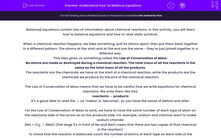# Understand How To Balance Equations

In this worksheet, students will learn how to balance chemical reaction equations including the use of state symbols.Key stage:  KS 4

Year:  GCSE

GCSE Boards:   AQA, AQA Trilogy, AQA Synergy, OCR 21st Century, OCR Gateway, Pearson Edexcel, Eduqas,

Difficulty level:#### Worksheet Overview

Balanced equations contain lots of information about chemical reactions. In this activity, you will learn how to balance equations and how to read state symbols.

When a chemical reaction happens, we take something, pull its atoms apart, then put them back together in a different pattern. The atoms at the start and at the end are the same - they're just joined together in a different way.

This idea gives us something called the Law of Conservation of Mass:

No atoms are made or destroyed during a chemical reaction. The total mass of all the reactants is the same as the total mass of all the products.

The reactants are the chemicals we have at the start of a chemical reaction, while the products are the chemicals we produce by the end of the chemical reaction.

The Law of Conservation of Mass means that we have to be careful how we write equations for chemical reactions. We write them like this:

reactants → products

It's a good idea to read the → as 'makes' or 'becomes', so you have the sense of before and after.

For the Law of Conservation of Mass to work, we have to have the same number of each type of atom on the reactants side of the arrow as on the products side. For example, sodium and chlorine react to make sodium chloride:

2Na + Cl2 → 2NaCl (the large 2's in front of Na and NaCl mean that there are two copies of that chemical in the reaction).

To check that the reaction is balanced, count the number of atoms of each type on each side of the reaction.

Na: before the reaction happens, there are 2Na, and after the reaction happens, there are 2Na.

Cl: before the reaction happens, there are 2Cl, and after the reaction happens, there are 2Cl.

No atoms have been made or destroyed, so the reaction is balanced.

If we need to balance an equation, we can't change the formulas of the chemicals we have, but we can change how many units of that chemical we use. So we were allowed to change NaCl to 2NaCl, but not to Na2Cl.

We can change the large numbers, but not the small ones.

Often, we add state symbols to each chemical, to tell us what state the chemical is in. There are four symbols you need to know:

Symbol Meaning
(s) Solid
(l) Liquid
(g) Gas
(aq) Aqueous (dissolved in water)

Sometimes, the equations are quite complicated, so we need a strategy. Here's what to do with an unbalanced equation:

CaCO3 + HCl → CaCl2 + H2O + CO2

First, find elements which only appear once on each side of the equation, and balance those compounds. Ignore oxygen for now because that is in several compounds.

There's one Ca in CaCO3, and one Ca in CaCl2 . So their numbers of formula units must be equal.

There's one H in HCl, but two H in H2O. So we need two units of HCl for each one unit of H2O.

There's one Cl in HCl, but two Cl in CaCl2. So we need two units of HCl for each one unit of CaCl2.

This means that balancing H and Cl needs the equation to be:

CaCO3 + 2HCl → CaCl2 + H2O + CO2

Then, once the easier elements are balanced, check the oxygens. There are three on the reactants side, and three on the products side, so that's OK. If you have elements which appear as pure elements (for example, H2 or O2) leave them until last.

Finally, add state symbols. Calcium carbonate is solid, hydrochloric acid is aqueous, calcium chloride dissolves in water, water is liquid and carbon dioxide is a gas - so we end up with.

CaCO3 (s) + 2HCl (aq)→ CaCl2 (aq) + H2O (l)+ CO2 (g)

That's it!

So, to summarise, to balance equations, make sure that there are the same number of atoms of each element on each side of the equation.

As long as you work through these gradually and systematically, it will work out fine.

Are you ready to have a go at some questions now?

### What is EdPlace?

We're your National Curriculum aligned online education content provider helping each child succeed in English, maths and science from year 1 to GCSE. With an EdPlace account you’ll be able to track and measure progress, helping each child achieve their best. We build confidence and attainment by personalising each child’s learning at a level that suits them.

Get started